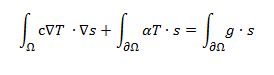Hello,

I have to formulate this equation in sfepy:So with \partialOmega = gamma:

"""dw_laplace.i.Omega(c,s, t ) + dw_volume_dot.i.Gamma( h , t, s)

=dw_surface_dot.i.Gamma(g, s )"""

My question is: alpha and g are constants of the surface of the airfoil, i have two files that contains the values of them for each point of the surface (we are in a 2D problem).

But i don't know how to define h and alpha in the configuration file as variables of the domain gamma with some ebcs conditions ?